September 29, 2023

Mensuration is a branch of mathematics that deals with the study of different geometrical shapes, their perimeter, area , surface area, curved surface area,  volume  etc. Basically,  there are two type of geometric shapes (i) 2D shapes   (ii) 3D shapes

2D shapes are : circle, square, rectangle, square , parallelogram, rhombus etc.

3D shapes are : cube , cylinder, cone , cuboid, sphere , prism , pyramid , cone etc.

Now let’s learn all the important mensuration formulas involving 2D and 3D shapes. Using this mensuration formulas list, it will be easy to solve the mensuration problems.

Contents

# Mensuration formulas for 2D -shapes:​

 Name Figure Area Perimeter Rectangle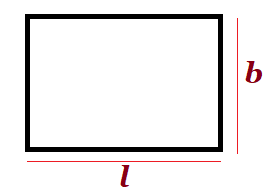l= length,   b=breadth Squarea= side ,   d= diagonal If d is given , then 4 x side= 4a Triangle (scalene)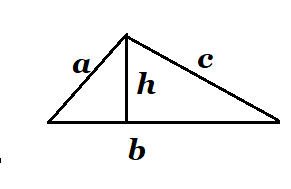b =base,    h=  height or altitude of a triangle (i) (ii)  Heron’s Formula a+b+c Equilateral triangle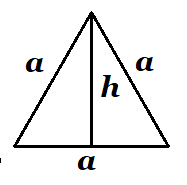a= side , h= height or  altitude (i) (ii) 3a Quadrilateral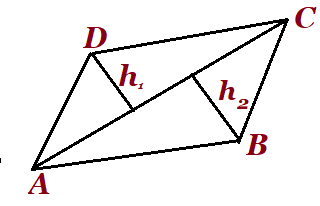AC=diagonals altitudes on AC from the vertices D and B respectively.(i)    (ii) product of diagonals x sin of the angle between them AB+BC+CD+AD Parallelograma and b be the lengths of parallel sides and h be the height (i)Area= base x  height (ii) area=   , is the angle between the sides of the parallelogram 2(a+b) Rhombus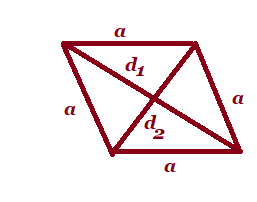a=each equal sides  ,                                          and   are the diagonals 4a Trapezium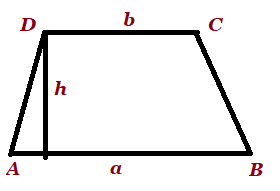a, b are parallel sides h is the perpendicular distance between parallel sides AB+BC+CD+DA Circle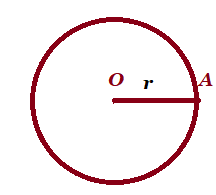r=radius , circumference= Semi-circle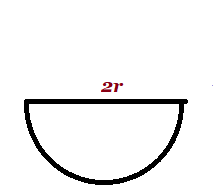r =radius Sector of a circleo  centre   , r= radius l=length of arc AB,  = angle of the sector (i) (ii) l+2r Regular hexagon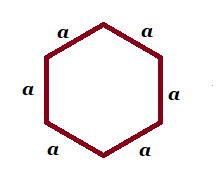a= each of the equal side 6a Regular octagon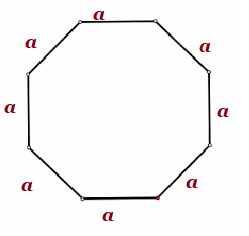a= each of the equal side 8a

## Mensuration formulas for 3D -shapes:

 Name Figure Volume Lateral /Curved surface area Total surface Area Cube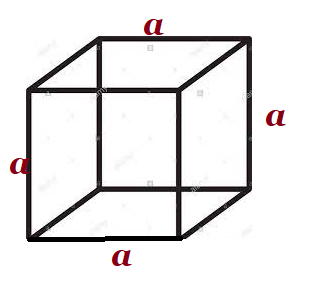a=side/edge cuboid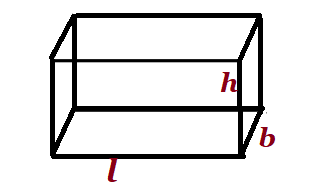l=length, b=breadth,  h=height lbh Right circular cylinderr= radius of base  h=height Right triangular prism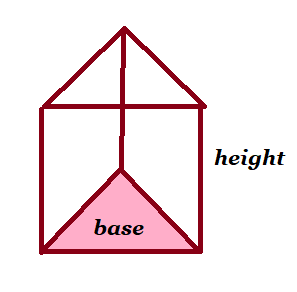area of base x height perimeter of base x height lateral surface area+2(area of base) Sphere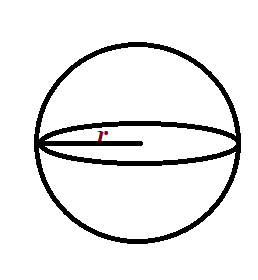r=radius Hemisphere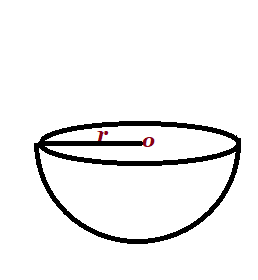r= radius Pyramid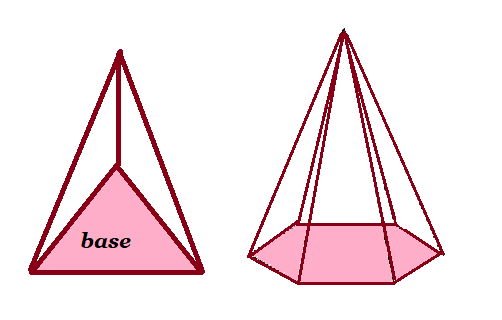l= slant height base x height perimeter of base x slant height lateral surface area+base area Cone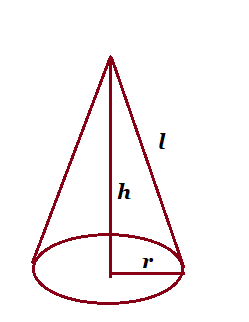l=slant height,   h =height , r= radius of base area of base x height= Frustum of a cone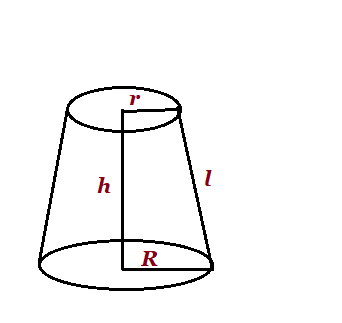lateral surface area+